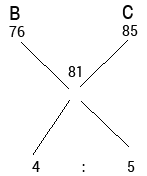# Sample Aptitude Questions of Maruti

1. There are 6 consecutive odd numbers. The difference between the square of the average of the first three numbers and the square of the average of the last three numbers is 288. What is the last odd number?
1. 31
2. 27
3. 29
4. 25
5. 33

Let the 6 consecutive odd no.’s are:
X, X+2, X+4, X+6, X+8, X+10
Avg. of 1st three no’s is X+2.
Avg. of Last three no’s is X+8.
Given that (X+8)2-(X+2)2=288
X=19
Last Odd no. is X+10= 29.
2. In a bag there are 6 red balls and 9 green balls. Two balls are drawn at random, what is the probability that at least one of the balls drawn is red?
1. 29/35
2. 7/15
3. 23/35
4. 2/5
5. 19/35

Probability of atleast one of the balls drawn is red= 1- (9/15) * (8/14)=23/35.
1. A started a business with an investment of Rs. 28,000. After 5 months from the start of the business, B and C joined with Rs. 24,000and Rs. 32,000 respectively and withdrew Rs. 8000 from the business. If the difference between A’s share and B’s share in the annual profit is Rs. 2,400, what was the annual profit received?
1. Rs. 15,600
2. Rs. 14,400
3. Rs. 14,040
4. Rs. 15,360
5. Rs. 13,440

Equivalent Contribution of A= 28000 * 5+20000 * 7= 280000
Equivalent Contribution of B= 24000 * 7= 168000
Equivalent Contribution of C= 32000 * 7= 224000
Let total profit be X.
Given that,
280000X/672000 – 168000X/672000=2400
112000/672000 * X=2400
or X=2400 * 672/112
X=14400
2. At present, Ami’s age is twice Dio’s age and Cami is two years older than Ami. Two years ago, the respective ratio between Dio’s age at that time and Cami’s age at that time was 4 : 9. What will be Ami’s age four years hence?
1. 40 years
2. 30 years
3. 42 years
4. 36 years
5. 48 years

A = 2D; C = A+2 = 2D+2
Given, D-2/(2D+2)-2 = 4/9
Solving, D = 18 Years and A = 36+4 = 40 years.
3. Three Science classes A, B and C take a Life Science test. The average score of students of class A is83. The average score of students class B is 76. The average score of class C is 85. The average score of class A and 8 is 79 and average score of class B and C is 81. Then the average score. Of classes A, B and C is
1. 80
2. 80.5
3. 81
4. 81.5
5. None of these

T.I.=30,000, N.T.I.=18000
Taxable 12000, Income Tax = 25% of Taxable Income
= 1/4 ×: 12000 = RS.3000A:B:C = 3:4:5
Sum of A+B+C = 3*83+4*76+5*85 = 978
Average score = 978/12 = 81.5
4. A hemispherical bowl of internal diameter 54 cm contains a liquid. The liquid is to be filled in cylindrical bottles of radius 3 cm and height 9 cm. How many bottles are required to empty the bowl?
1. 221
2. 343
3. 81
4. 243
5. None of these

Area of a hemispherical bowl = 2/3 ×π ×r3
Area of a cylinder = πr2h
Area of a cylinder = n × Area of a hemispherical bowl
2/3 ×π ×273 = n ×π×32×9
we get n = 162
DIRECTIONS for the questions 7 & 9 : What approximate value will come in place of question mark (?) in the given question?( You are not expected to calculate the exact value.)
5. 26.00 - 154.001/6.995 = ?
1. 4
2. 18
3. 9
4. 10
5. 14

26 – (154/7) = 4
6. 17.995/3.01 + 104.001/12.999 = ?
1. 11
2. 20
3. 23
4. 14
5. 17

18/3 + 104/13 = 14
7. 3/5 of 4/7 of 7/9 of 425 = ?
1. 121
2. 110
3. 118
4. 113
5. 124